# Selecting rows in pandas DataFrame based on conditions

Let’s see how to Select rows based on some conditions in Pandas DataFrame.

### Selecting rows based on particular column value using `'>', '=', '=', '<=', '!=' `operator.

Code #1 : Selecting all the rows from the given dataframe in which ‘Percentage’ is greater than 80 using basic method.

 `# importing pandas ` `import` `pandas as pd ` ` `  `record ``=` `{ ` ` `  ` ``'Name'``: [``'Ankit'``, ``'Amit'``, ``'Aishwarya'``, ``'Priyanka'``, ``'Priya'``, ``'Shaurya'` `], ` ` ``'Age'``: [``21``, ``19``, ``20``, ``18``, ``17``, ``21``], ` ` ``'Stream'``: [``'Math'``, ``'Commerce'``, ``'Science'``, ``'Math'``, ``'Math'``, ``'Science'``], ` ` ``'Percentage'``: [``88``, ``92``, ``95``, ``70``, ``65``, ``78``] } ` ` `  `# create a dataframe ` `dataframe ``=` `pd.DataFrame(record, columns ``=` `[``'Name'``, ``'Age'``, ``'Stream'``, ``'Percentage'``]) ` ` `  `print``(``"Given Dataframe :\n"``, dataframe)  ` ` `  `# selecting rows based on condition ` `rslt_df ``=` `dataframe[dataframe[``'Percentage'``] > ``80``] ` ` `  `print``(``'\nResult dataframe :\n'``, rslt_df) `

Output :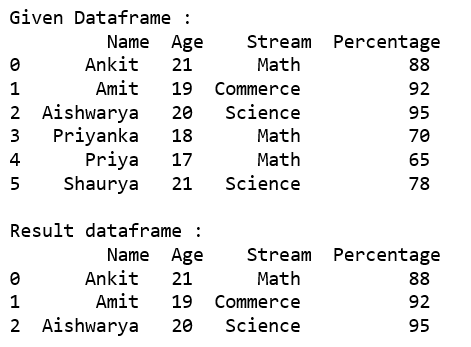Code #2 : Selecting all the rows from the given dataframe in which ‘Percentage’ is greater than 80 using `loc[]`.

 `# importing pandas ` `import` `pandas as pd ` ` `  `record ``=` `{ ` `  ``'Name'``: [``'Ankit'``, ``'Amit'``, ``'Aishwarya'``, ``'Priyanka'``, ``'Priya'``, ``'Shaurya'` `], ` `  ``'Age'``: [``21``, ``19``, ``20``, ``18``, ``17``, ``21``], ` `  ``'Stream'``: [``'Math'``, ``'Commerce'``, ``'Science'``, ``'Math'``, ``'Math'``, ``'Science'``], ` `  ``'Percentage'``: [``88``, ``92``, ``95``, ``70``, ``65``, ``78``]} ` ` `  `# create a dataframe ` `dataframe ``=` `pd.DataFrame(record, columns ``=` `[``'Name'``, ``'Age'``, ``'Stream'``, ``'Percentage'``]) ` ` `  `print``(``"Given Dataframe :\n"``, dataframe)  ` ` `  `# selecting rows based on condition ` `rslt_df ``=` `dataframe.loc[dataframe[``'Percentage'``] > ``80``] ` ` `  `print``(``'\nResult dataframe :\n'``, rslt_df) `

Output :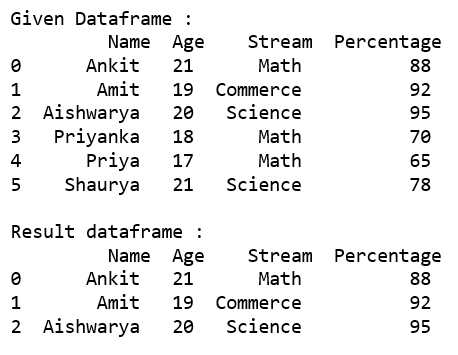Code #3 : Selecting all the rows from the given dataframe in which ‘Percentage’ is not equal to 95 using `loc[]`.

 `# importing pandas ` `import` `pandas as pd ` ` `  `record ``=` `{ ` `  ``'Name'``: [``'Ankit'``, ``'Amit'``, ``'Aishwarya'``, ``'Priyanka'``, ``'Priya'``, ``'Shaurya'` `], ` `  ``'Age'``: [``21``, ``19``, ``20``, ``18``, ``17``, ``21``], ` `  ``'Stream'``: [``'Math'``, ``'Commerce'``, ``'Science'``, ``'Math'``, ``'Math'``, ``'Science'``], ` `  ``'Percentage'``: [``88``, ``92``, ``95``, ``70``, ``65``, ``78``]} ` ` `  `# create a dataframe ` `dataframe ``=` `pd.DataFrame(record, columns ``=` `[``'Name'``, ``'Age'``, ``'Stream'``, ``'Percentage'``]) ` ` `  `print``(``"Given Dataframe :\n"``, dataframe)  ` ` `  `# selecting rows based on condition ` `rslt_df ``=` `dataframe.loc[dataframe[``'Percentage'``] !``=` `95``] ` ` `  `print``(``'\nResult dataframe :\n'``, rslt_df) `

Output :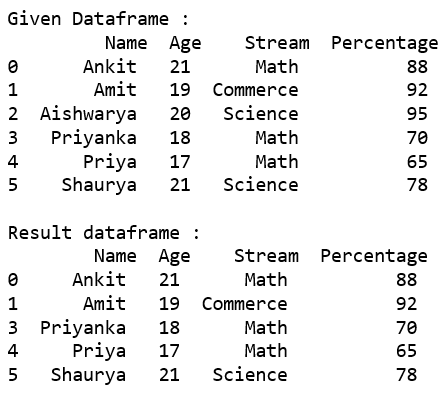### Selecting those rows whose column value is present in the list using `isin()` method of the dataframe.

Code #1 : Selecting all the rows from the given dataframe in which ‘Stream’ is present in the options list using basic method.

 `# importing pandas ` `import` `pandas as pd ` ` `  `record ``=` `{ ` `  ``'Name'``: [``'Ankit'``, ``'Amit'``, ``'Aishwarya'``, ``'Priyanka'``, ``'Priya'``, ``'Shaurya'` `], ` `  ``'Age'``: [``21``, ``19``, ``20``, ``18``, ``17``, ``21``], ` `  ``'Stream'``: [``'Math'``, ``'Commerce'``, ``'Science'``, ``'Math'``, ``'Math'``, ``'Science'``], ` `  ``'Percentage'``: [``88``, ``92``, ``95``, ``70``, ``65``, ``78``]} ` ` `  `# create a dataframe ` `dataframe ``=` `pd.DataFrame(record, columns ``=` `[``'Name'``, ``'Age'``, ``'Stream'``, ``'Percentage'``]) ` ` `  `print``(``"Given Dataframe :\n"``, dataframe)  ` ` `  `options ``=` `[``'Math'``, ``'Commerce'``] ` ` `  `# selecting rows based on condition ` `rslt_df ``=` `dataframe[dataframe[``'Stream'``].isin(options)] ` ` `  `print``(``'\nResult dataframe :\n'``, rslt_df) `

Output :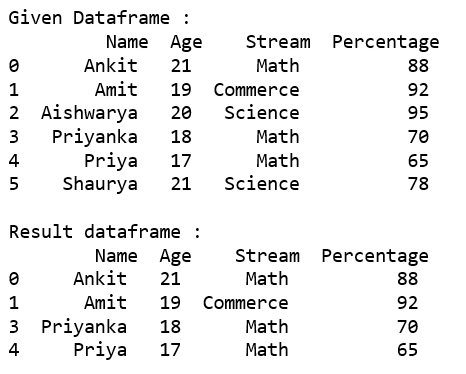Code #2 : Selecting all the rows from the given dataframe in which ‘Stream’ is present in the options list using `loc[]`.

 `# importing pandas ` `import` `pandas as pd ` ` `  `record ``=` `{ ` `  ``'Name'``: [``'Ankit'``, ``'Amit'``, ``'Aishwarya'``, ``'Priyanka'``, ``'Priya'``, ``'Shaurya'` `], ` `  ``'Age'``: [``21``, ``19``, ``20``, ``18``, ``17``, ``21``], ` `  ``'Stream'``: [``'Math'``, ``'Commerce'``, ``'Science'``, ``'Math'``, ``'Math'``, ``'Science'``], ` `  ``'Percentage'``: [``88``, ``92``, ``95``, ``70``, ``65``, ``78``]} ` ` `  `# create a dataframe ` `dataframe ``=` `pd.DataFrame(record, columns ``=` `[``'Name'``, ``'Age'``, ``'Stream'``, ``'Percentage'``]) ` ` `  `print``(``"Given Dataframe :\n"``, dataframe)  ` ` `  `options ``=` `[``'Math'``, ``'Commerce'``] ` ` `  `# selecting rows based on condition ` `rslt_df ``=` `dataframe.loc[dataframe[``'Stream'``].isin(options)] ` ` `  `print``(``'\nResult dataframe :\n'``, rslt_df) `

Output :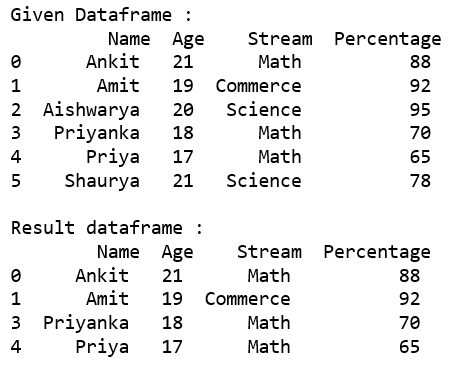Code #3 : Selecting all the rows from the given dataframe in which ‘Stream’ is not present in the options list using `.loc[]`.

 `# importing pandas ` `import` `pandas as pd ` ` `  `record ``=` `{ ` `  ``'Name'``: [``'Ankit'``, ``'Amit'``, ``'Aishwarya'``, ``'Priyanka'``, ``'Priya'``, ``'Shaurya'` `], ` `  ``'Age'``: [``21``, ``19``, ``20``, ``18``, ``17``, ``21``], ` `  ``'Stream'``: [``'Math'``, ``'Commerce'``, ``'Science'``, ``'Math'``, ``'Math'``, ``'Science'``], ` `  ``'Percentage'``: [``88``, ``92``, ``95``, ``70``, ``65``, ``78``]} ` ` `  `# create a dataframe ` `dataframe ``=` `pd.DataFrame(record, columns ``=` `[``'Name'``, ``'Age'``, ``'Stream'``, ``'Percentage'``]) ` ` `  `print``(``"Given Dataframe :\n"``, dataframe)  ` ` `  `options ``=` `[``'Math'``, ``'Science'``] ` ` `  `# selecting rows based on condition ` `rslt_df ``=` `dataframe.loc[~dataframe[``'Stream'``].isin(options)] ` ` `  `print``(``'\nresult dataframe :\n'``, rslt_df) `

Output :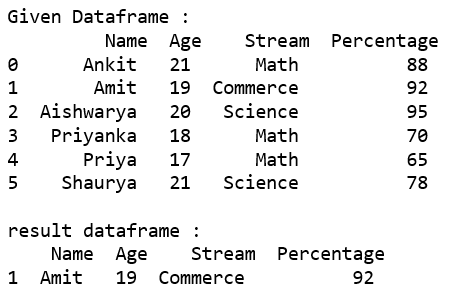### Selecting rows based on multiple column conditions using `'&'` operator.

Code #1 : Selecting all the rows from the given dataframe in which ‘Age’ is equal to 21 and ‘Stream’ is present in the options list using basic method.

 `# importing pandas ` `import` `pandas as pd ` ` `  `record ``=` `{ ` `  ``'Name'``: [``'Ankit'``, ``'Amit'``, ``'Aishwarya'``, ``'Priyanka'``, ``'Priya'``, ``'Shaurya'` `], ` `  ``'Age'``: [``21``, ``19``, ``20``, ``18``, ``17``, ``21``], ` `  ``'Stream'``: [``'Math'``, ``'Commerce'``, ``'Science'``, ``'Math'``, ``'Math'``, ``'Science'``], ` `  ``'Percentage'``: [``88``, ``92``, ``95``, ``70``, ``65``, ``78``]} ` ` `  `# create a dataframe ` `dataframe ``=` `pd.DataFrame(record, columns ``=` `[``'Name'``, ``'Age'``, ``'Stream'``, ``'Percentage'``]) ` ` `  `print``(``"Given Dataframe :\n"``, dataframe)  ` ` `  `options ``=` `[``'Math'``, ``'Science'``] ` ` `  `# selecting rows based on condition ` `rslt_df ``=` `dataframe[(dataframe[``'Age'``] ``=``=` `21``) & ` `          ``dataframe[``'Stream'``].isin(options)] ` ` `  `print``(``'\nResult dataframe :\n'``, rslt_df) `

Output :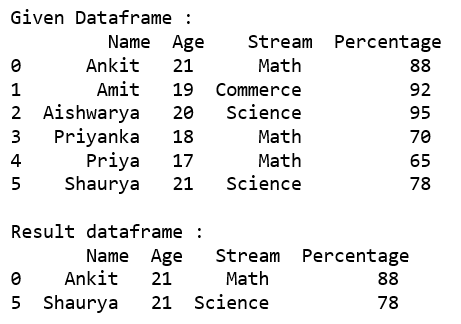Code #2 : Selecting all the rows from the given dataframe in which ‘Age’ is equal to 21 and ‘Stream’ is present in the options list using .loc[].

 `# importing pandas ` `import` `pandas as pd ` ` `  `record ``=` `{ ` `  ``'Name'``: [``'Ankit'``, ``'Amit'``, ``'Aishwarya'``, ``'Priyanka'``, ``'Priya'``, ``'Shaurya'` `], ` `  ``'Age'``: [``21``, ``19``, ``20``, ``18``, ``17``, ``21``], ` `  ``'Stream'``: [``'Math'``, ``'Commerce'``, ``'Science'``, ``'Math'``, ``'Math'``, ``'Science'``], ` `  ``'Percentage'``: [``88``, ``92``, ``95``, ``70``, ``65``, ``78``]} ` ` `  `# create a dataframe ` `dataframe ``=` `pd.DataFrame(record, columns ``=` `[``'Name'``, ``'Age'``, ``'Stream'``, ``'Percentage'``]) ` ` `  `print``(``"Given Dataframe :\n"``, dataframe)  ` ` `  `options ``=` `[``'Math'``, ``'Science'``] ` ` `  `# selecting rows based on condition ` `rslt_df ``=` `dataframe.loc[(dataframe[``'Age'``] ``=``=` `21``) & ` `              ``dataframe[``'Stream'``].isin(options)] ` ` `  `print``(``'\nResult dataframe :\n'``, rslt_df) `

Output :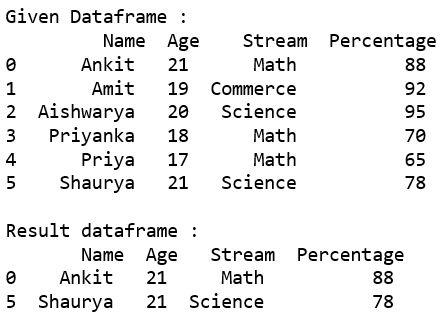My Personal Notes arrow_drop_upCheck out this Author's contributed articles.

If you like GeeksforGeeks and would like to contribute, you can also write an article using contribute.geeksforgeeks.org or mail your article to contribute@geeksforgeeks.org. See your article appearing on the GeeksforGeeks main page and help other Geeks.

Please Improve this article if you find anything incorrect by clicking on the "Improve Article" button below.

Article Tags :

34

Please write to us at contribute@geeksforgeeks.org to report any issue with the above content.